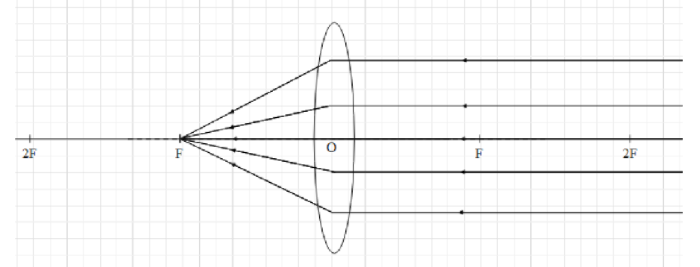# Why is a convex lens called a converging lens?

Last updated date: 27th Jan 2023
Total views: 234.3k
Views today: 2.28kVerified
234.3k+ views
Hint:Convex lens is a spherical lens that is pointed towards the end (it is circumference) and it bulges outwards at the middle. When the rays of light coming from an object placed at infinite distance (very far) from the lens, pass through the convex lens they converge at a point after they are refracted.
A lens is a material of some refractive index, which helps in refracting the rays of light that pass through it. If we place an object in front of a lens, then the rays of light coming from the object refract when they pass through the lens. As a result, the original path of the light rays changes and the appear to be originating from a different point other than the position of the object.

Hence, we say that an image is formed at that point.
Convex lens is a type of lens that is pointed towards the end (it is circumference) and it bulges outwards at the middle (as shown the figure). Both the surfaces of a convex lens is spherical.

Let us understand the features or properties of a convex lens.

When the rays of light coming from an object placed at infinite distance (very far) from the lens, pass through the convex lens they converge at a point after they are refracted. As a result, the image is formed on the side of the lens.Since a convex lens converges the lights rays passing through, at a point, it is called a converging lens.

Note:There exist another type of spherical lens called concave lens. We can say that a concave lens is opposite to a convex lens. It widens towards the end and shrinks in the middle.

When parallel light rays pass through a concave lens, they diverge in all directions and appear to be originating from a point on the side of the lens. Hence, a concave lens is called a diverging lens.